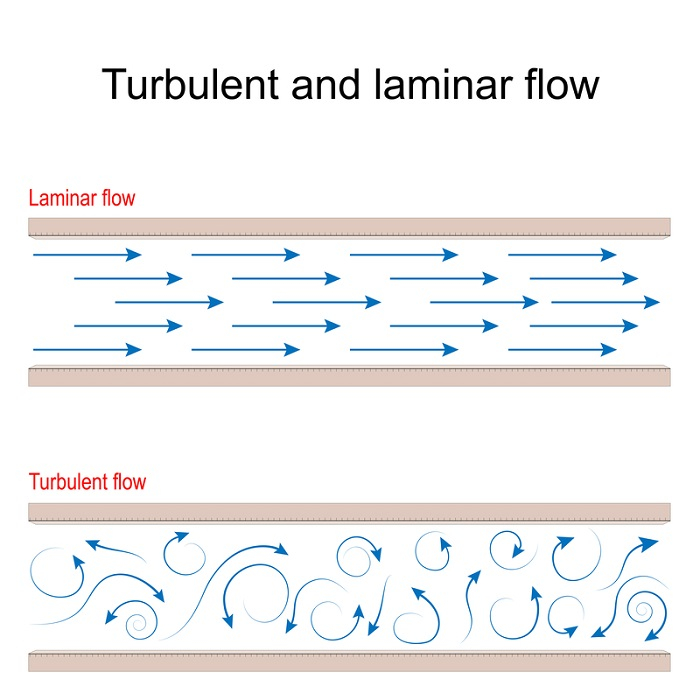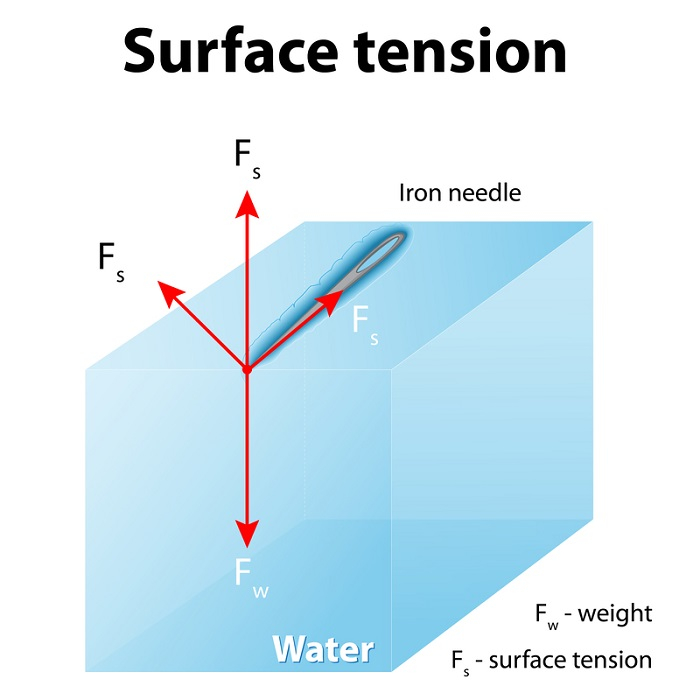# Mechanical Properties of Fluids

## Introduction

The mechanical properties of fluids are a branch of physics that states about the mechanics of the fluids, it includes the liquid, gas and the plasmas. Fluids are incompressible which means the density of the liquid is not dependent on the variation in the pressure and remains as constant. They also count as the most non-viscous. It means, that two liquid surfaces that came in contact are not pressing any kind of tangential force on one another.

## What is Fluid?

In the field of chemistry, the term fluid can be referred to any liquid or gas that cannot sustain a tangential or shearing force, when it is in the resting situation and it also went through a continuous change of shape depending on the subject such as stress. In short, it is a substance that can flow.

In other words, it can flow only when an external force is applied to the substance. For example, a gas can be oxygen, hydrogen, in liquids, such as petrol, sulphuric acid, and water. It can be classified into four major types and it depends on the flow. They are- steady or unsteady, compressible or the opposite, vicious or the exact opposite of that, and lastly, rotational or irrotational.

## Mechanical Properties of Fluid

The branch of science that talks about mechanical properties, is called hydrostatics. A fluid is a substance that has no specific shape and as liquid and gas can flow freely they are called fluids. They can yield easily to any kind of external pressure (learncbse, 2022). The gs part of the fluid refers to the air of the atmosphere and all other kinds of air that are part of chemistry.

Fluids can show the gravitational effect in a more specific manner than solids. The latter one has the attraction of the particles, those measures counteracting the effect of gravity.

## What is Streamline Flow?Figure 1: Streamline Flow

The streamline refers to a curve of the tangent. At any point, it provides the direction of the velocity of the fluid. In an electrical or mechanical field, it has an analogous relation to the line of force.

The streamline can show the original pathway of a fluid particle. When the flow is steady it is called the laminar flow. The main thing about this is that two of the streamlines can never cross each other. If it happens then one fluid particle can go one way or the other at that point. However, the flow will be unsteady.

## Tube of Flow

The tube of flow is a bundle of streamline. A tubular region that is enclosed by a boundary that consists of streamlines, is called the tube of flow. The main thing about this is that no fluid can cross the boundary that is made by the tube of flow and its behavior is exactly like a pipe.

## Surface Tension and ViscosityFigure 2: A needle stays atop the liquid because of surface tension. If the needle were placed point down on the surface, its weight acting on a smaller area would break the surface, and it would sink.

The amount of force per unit length that is necessary for the process of deforming the interfacial surface is known as the surface tension. It can be represented by the Greek letter sigma. The surface tension always depends upon the surface that the liquid or the solid is in touch with. If the surface tension of oil, water, mercury, or anything else is measured, then it can be assumed that the contacting fluid is nothing but the air.

The viscosity can be defined as the ratio of the shearing stress that is between the adjacent layers of the fluids. It is a property of a fluid that can be the reason behind the resist flow of the fluid. The higher the viscosity is the higher the resting flow will be. The viscosity of the fluids that are known to everyone, is majorly independent to the external force.

## Bernoulli’s Principle

Bernoulli’s Principle states that the total energy of the water remains constant. That is the reason when the water flow increases systemically the pressure decreases eventually. When it starts to flow in a hydraulic system the pressure drops constantly and when the pressure starts rising, the flow must be stopped.

This can be expressed as -

## Pressure

The term fluid pressure can be explained as the force exerted normally at a unit area of the surface of the fluid. The pressure is known as the vapor pressure of the liquid. Or it can be the pressure that can convert the liquid into vapor.

## Conclusion

The mechanical property of Fluids is such as viscosity and surface tension. From the mechanical angle, the fluid is a kind of substance that never supports the shear stress. That is the main reason why one fluid takes the shape of its vessel when it is in a resting phase.

## (FAQs)

Q1. What is a Venturi-meter?

Ans. The venturi-meter is an instrument that is used to give an increase in the flow velocity by a converging section of a pipe and a corresponding drop of pressure occurs so that the flow rate can be deduced.

Q2. What is the effect of the fluid pressure?

Ans. Two factors can determine the fluid pressure. They are the depth of the fluid and the intensity.

Q3. What are the properties of the fluid?

Ans. Fluids have 4 main properties; they are temperature, density, pressure, and the specific enthalpy. They are the thermodynamic properties of the fluids.

Q4. What are the characteristics of the liquid?

Ans. Major characteristics that can define the liquid are- it does not have a definite shape, it takes the exact shape of the container, and it has a definite volume. The particles of the liquids are free, so they can move over each other, however, they still maintain the attraction for each other.